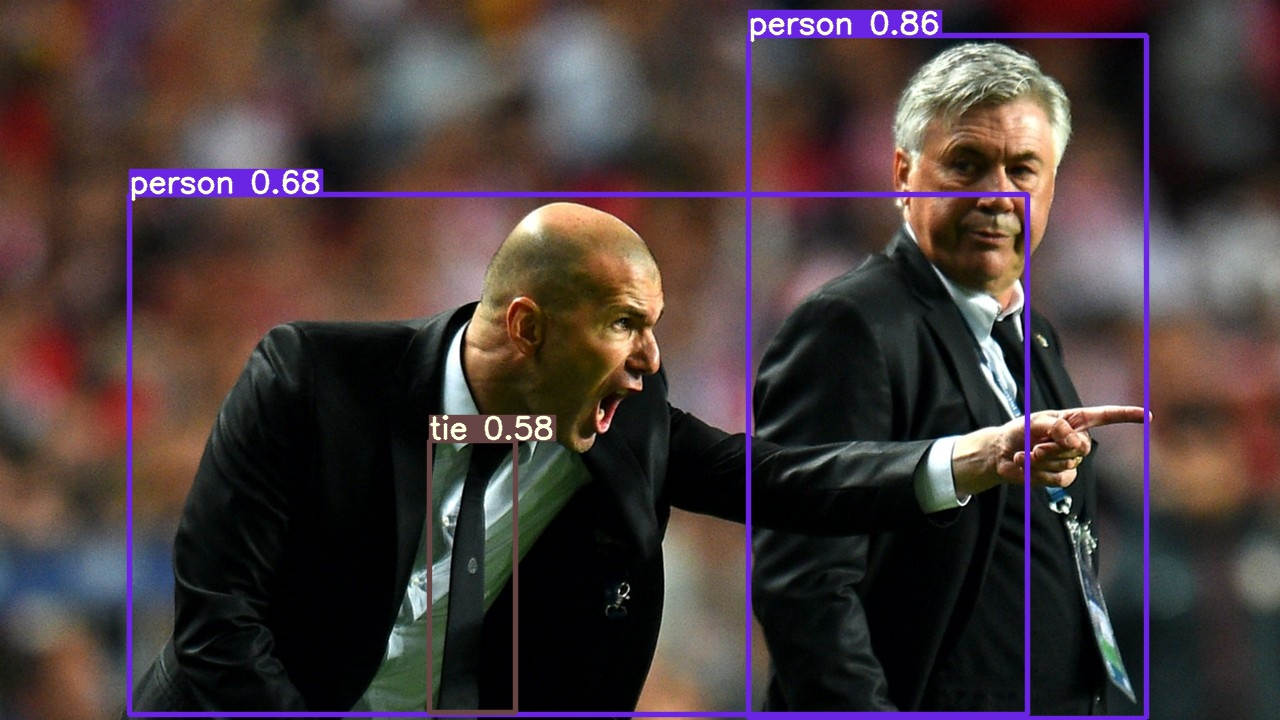Test-Time Augmentation (TTA)

📚 This guide explains how to use Test Time Augmentation (TTA) during testing and inference for improved mAP and Recall with YOLOv5 🚀.

Before You Start

Clone this repo and install requirements.txt dependencies, including Python>=3.8 and PyTorch>=1.7.

git clone https://github.com/ultralytics/yolov5 # clone repo
cd yolov5
pip install -r requirements.txt # install requirements.txt

Test Normally

Before trying TTA we want to establish the baseline performance by testing under default settings. This command tests YOLOv5x on COCO val2017 at image size 640 pixels. yolov5x.pt is the largest and most accurate model available. Other options are yolov5s.pt, yolov5m.pt and yolov5l.pt, or you own checkpoint from training a custom dataset ./weights/best.pt. For details on all available models please see our README table.

\$ python test.py --weights yolov5x.pt --data coco.yaml --img 640

Output:

Namespace(augment=False, batch_size=32, conf_thres=0.001, data='./data/coco.yaml', device='', img_size=640, iou_thres=0.65, save_json=True, save_txt=False, single_cls=False, task='val', verbose=False, weights=['yolov5x.pt'])
Using CUDA device0 _CudaDeviceProperties(name='Tesla P100-PCIE-16GB', total_memory=16280MB)

Fusing layers... Model Summary: 284 layers, 8.89222e+07 parameters, 0 gradients
Scanning labels ../coco/labels/val2017.cache (4952 found, 0 missing, 48 empty, 0 duplicate, for 5000 images): 5000it [00:00, 17761.74it/s]
Class      Images     Targets           P           R      mAP@.5  mAP@.5:.95: 100% 157/157 [02:34<00:00,  1.02it/s]
all       5e+03    3.63e+04       0.409       0.754       0.669       0.476
Speed: 23.6/1.6/25.2 ms inference/NMS/total per 640x640 image at batch-size 32 < ---------- baseline speed

COCO mAP with pycocotools... saving detections_val2017__results.json...
Average Precision  (AP) @[ IoU=0.50:0.95 | area=   all | maxDets=100 ] = 0.492 < ---------- baseline mAP
Average Precision  (AP) @[ IoU=0.50      | area=   all | maxDets=100 ] = 0.676
Average Precision  (AP) @[ IoU=0.75      | area=   all | maxDets=100 ] = 0.534
Average Precision  (AP) @[ IoU=0.50:0.95 | area= small | maxDets=100 ] = 0.318
Average Precision  (AP) @[ IoU=0.50:0.95 | area=medium | maxDets=100 ] = 0.541
Average Precision  (AP) @[ IoU=0.50:0.95 | area= large | maxDets=100 ] = 0.633
Average Recall     (AR) @[ IoU=0.50:0.95 | area=   all | maxDets=  1 ] = 0.376
Average Recall     (AR) @[ IoU=0.50:0.95 | area=   all | maxDets= 10 ] = 0.616
Average Recall     (AR) @[ IoU=0.50:0.95 | area=   all | maxDets=100 ] = 0.670 < ---------- baseline mAR
Average Recall     (AR) @[ IoU=0.50:0.95 | area= small | maxDets=100 ] = 0.493
Average Recall     (AR) @[ IoU=0.50:0.95 | area=medium | maxDets=100 ] = 0.723
Average Recall     (AR) @[ IoU=0.50:0.95 | area= large | maxDets=100 ] = 0.812

Test with TTA

Append --augment to any existing test.py command to enable TTA, and increase the image size by about 30% for improved results. Note that inference with TTA enabled will typically take about 2-3X the time of normal inference as the images are being left-right flipped and processed at 3 different resolutions, with the outputs merged before NMS. Part of the speed decrease is simply due to larger image sizes (832 vs 640), while part is due to the actual TTA operations.

\$ python test.py --weights yolov5x.pt --data coco.yaml --img 832 --augment

Output:

Namespace(augment=True, batch_size=32, conf_thres=0.001, data='./data/coco.yaml', device='', img_size=832, iou_thres=0.65, save_json=True, save_txt=False, single_cls=False, task='val', verbose=False, weights=['yolov5x.pt'])
Using CUDA device0 _CudaDeviceProperties(name='Tesla P100-PCIE-16GB', total_memory=16280MB)

Fusing layers... Model Summary: 284 layers, 8.89222e+07 parameters, 0 gradients
Scanning labels ../coco/labels/val2017.cache (4952 found, 0 missing, 48 empty, 0 duplicate, for 5000 images): 5000it [00:00, 17064.47it/s]
Class      Images     Targets           P           R      mAP@.5  mAP@.5:.95: 100% 157/157 [07:54<00:00,  3.02s/it]
all       5e+03    3.63e+04       0.309       0.807       0.682       0.492
Speed: 85.1/3.6/88.7 ms inference/NMS/total per 832x832 image at batch-size 32 < ---------- reduced speed

COCO mAP with pycocotools... saving detections_val2017__results.json...
...
Average Precision  (AP) @[ IoU=0.50:0.95 | area=   all | maxDets=100 ] = 0.508 < ---------- improved mAP
Average Precision  (AP) @[ IoU=0.50      | area=   all | maxDets=100 ] = 0.689
Average Precision  (AP) @[ IoU=0.75      | area=   all | maxDets=100 ] = 0.556
Average Precision  (AP) @[ IoU=0.50:0.95 | area= small | maxDets=100 ] = 0.346
Average Precision  (AP) @[ IoU=0.50:0.95 | area=medium | maxDets=100 ] = 0.557
Average Precision  (AP) @[ IoU=0.50:0.95 | area= large | maxDets=100 ] = 0.648
Average Recall     (AR) @[ IoU=0.50:0.95 | area=   all | maxDets=  1 ] = 0.385
Average Recall     (AR) @[ IoU=0.50:0.95 | area=   all | maxDets= 10 ] = 0.635
Average Recall     (AR) @[ IoU=0.50:0.95 | area=   all | maxDets=100 ] = 0.696 < ---------- improved mAR
Average Recall     (AR) @[ IoU=0.50:0.95 | area= small | maxDets=100 ] = 0.536
Average Recall     (AR) @[ IoU=0.50:0.95 | area=medium | maxDets=100 ] = 0.740
Average Recall     (AR) @[ IoU=0.50:0.95 | area= large | maxDets=100 ] = 0.826

Inference with TTA

detect.py TTA inference operates identically to test.py TTA: simply append --augment to any existing detect.py command:

\$ python detect.py --weights yolov5s.pt --img 832 --source ./inference/images/ --augment

Output:

Namespace(agnostic_nms=False, augment=True, classes=None, conf_thres=0.25, device='', img_size=832, iou_thres=0.45, output='inference/output', save_txt=False, source='./inference/images/', update=False, view_img=False, weights=['yolov5s.pt'])
Using CUDA device0 _CudaDeviceProperties(name='Tesla P100-PCIE-16GB', total_memory=16280MB)

Fusing layers... Model Summary: 140 layers, 7.45958e+06 parameters, 0 gradients
image 1/2 /yolov5/inference/images/bus.jpg: 832x640 4 persons, 1 buss, Done. (0.037s)
image 2/2 /yolov5/images/zidane.jpg: 512x832 2 persons, 3 ties, Done. (0.036s)
Results saved to inference/output
Done. (0.186s)PyTorch Hub TTA

TTA is automatically integrated into all YOLOv5 PyTorch Hub models, and can be accessed by passing augment=True at inference time.

import torch

# Model

# Images
dir = 'https://github.com/ultralytics/yolov5/raw/master/data/images/'
imgs = [dir + f for f in ('zidane.jpg', 'bus.jpg')]  # batch of images

# Inference
results = model(imgs, augment=True)  # TTA inference

Environments

YOLOv5 may be run in any of the following up-to-date verified environments (with all dependencies including CUDA/CUDNN, Python and PyTorch preinstalled):

StatusIf this badge is green, all YOLOv5 GitHub Actions Continuous Integration (CI) tests are currently passing. CI tests verify correct operation of YOLOv5 training (train.py), testing (test.py), inference (detect.py) and export (export.py) on MacOS, Windows, and Ubuntu every 24 hours and on every commit.